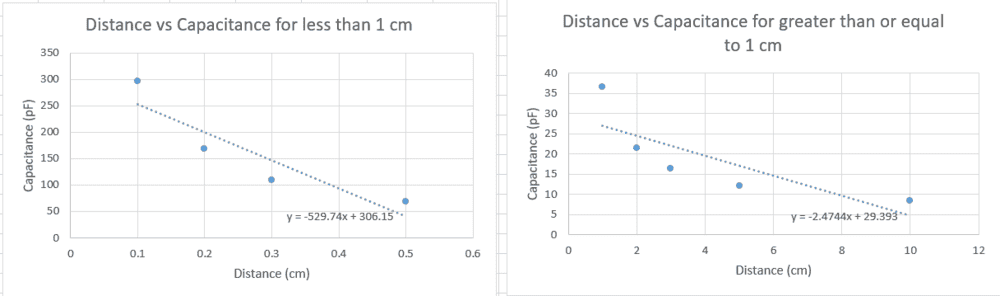# Capacitance between two plates

Homework Statement:
I had a physics lab where I measured the capacitance between two parallel plates. I was told to make two graphs of Capacitance vs. distance, one for i) d ≤ 1.0cm and another for ii) d ≥ 1.0cm. Then I was asked which data range (d>1cm or d< 1cm) better fits the parallel plate formula by comparing the graphs for each data range (see the pictures below). I was also asked to explain why it fits better in terms of the assumptions built into the derivation of the parallel plate formula.
Also, I am confused about which formula to use. I believe that I am supposed to use C=E0(A/d)

Also I believe that the assumptions of the parallel plate formula are that the plates are large and close together, so the charge is distributed uniformly over each plate (not entirely sure).
Because of this , I think that the graph for values of d less than 1 cm is more accurate, however i do not know how to explain this in terms of slope of the graph, or how to relate the equation y=-529+306 or how to explain this in terms of the assumptions built into the parallel plate formula.
Relevant Equations:
C=E0(A/d) (I am not sure if this is the correct equation to use)